# Methods and formulas for the Xbar chart in Xbar-R Chart

Select the method or formula of your choice.

## Plotted points

Each plotted point,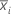, represents the mean of the observations for subgroup,.

### Notation

TermDescription
xijjth observation in the ith subgroup
ninumber of observations in subgroup i

## Center line

The center line represents the process mean (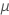). If you do not specify a historical value, then Minitab uses the average from your data,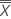, which is calculated as follows:

### Notation

TermDescription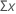sum of all individual observations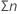total number of observations

## Control limits

### Lower control limit (LCL)

The value of the lower control limit for each subgroup, i, is calculated as follows:

### Upper control limit (UCL)

The value of the upper control limit for each subgroup, i, is calculated as follows:

### Notation

TermDescription
μprocess mean
kparameter for Test 1 (The default is 3.)
σprocess standard deviation
ninumber of observations in subgroup i
By using this site you agree to the use of cookies for analytics and personalized content.  Read our policy